Dijkstra’s algorithm is a simple, elegant graph algorithm, and one of my favourites.

The problem it solves is the following: given a graph with non-negative edge weights and a start node, how can we find the shortest path to other nodes in the graph?

Dijkstra’s algorithm takes a greedy approach to solve the problem. That is, instead of considering all possible combinations of edges, it arranges its steps such that it can iteratively make the best local choice, until it has constructed the shortest possible path to every other node.

In this case, the greedy algorithm is both correct and efficient.

### Dijkstra’s algorithm

1. Assign distance(start)=0, and distance(v)=infinity for other nodes.
2. Add all nodes to an “unvisited” set.
3. While there are unvisited nodes:
• Select the unvisited node v with the minimal distance value
• Remove v from the unvisited set
• For each edge (v, w):
• Set distance(w) = min { distance(w), distance(v) + weight(v,w) }

### Example of running Dijkstra’s algorithm manually

Consider the following graph with labelled edge weights. At the beginning, “start” is the only node with finite distance, so we mark it as visited and update the distances to “b” and “c”.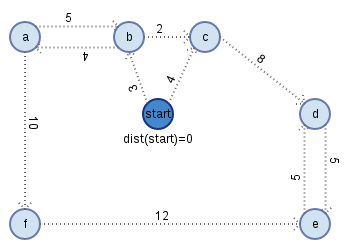Distances to unvisited nodes: b:3, c:4, others:infinity.

b is the closest unvisited node, so we mark it as visited, finalizing the shortest distance from start to b, and we update its neighbours’ distances.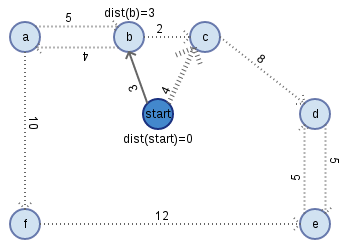Distances to unvisited nodes: c:min(4,3+2)=4, a:7, others:infinity.

c is now the closest unvisited node. We mark it as visited, finalizing distance(c)=4, and update the distance to its neighbour d.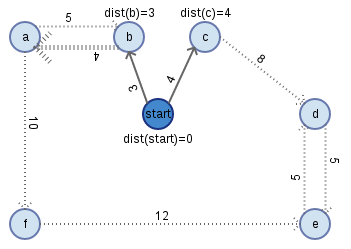Distances to unvisited nodes: a:7, d:12, others:infinity.

a is the closest, so we mark it as visited and update its neighbours’ distances.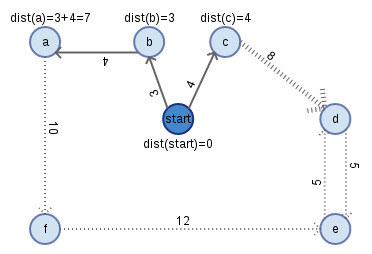Distances to unvisited nodes: d:12, f:17, others:infinity.

We mark d as visited and update its neighbours’ distances.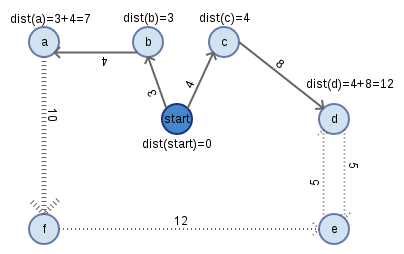Distances to unvisited nodes: e:17, f:17.

The distances are equal, so we can use either. We mark f as visited and update its neighbours’ distances.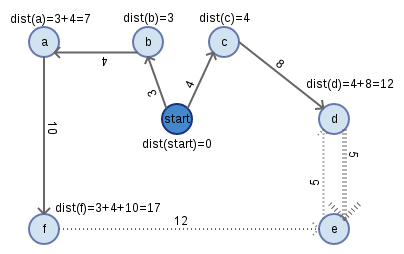Distances to unvisited nodes: e:min(17,17+12)=17.

We mark e as visited. There are no further unvisited nodes, so Dijkstra’s algorithm terminates, giving us the shortest path to every node.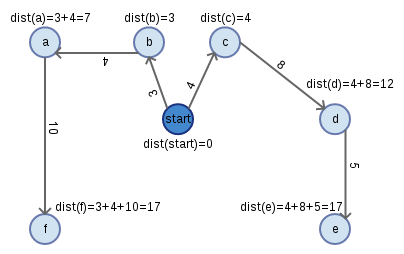### Proof of correctness

Base case: Dijkstra’s algorithm finds the shortest path to the start node s, distance(s)=0.

Inductive hypothesis: Assume that distance(v) is the shortest distance from the start node to v for each of the first k nodes that Dijkstra’s algorithm visits. Assume that the first k nodes that Dijkstra’s algorithm visits are the k closest nodes to the start node.

Inductive step: Let u be the (k+1)-th node visited. Then u is the unvisited node with the minimum distance value as set by the first k iterations of the while loop.

distance(u) = min { distance(w), distance(v) + weight(v,w) }, where v is the visited node that minimizes this value. Therefore, Dijkstra’s algorithm finds the shortest path P from the set of visited nodes to u that does not include other unvisited nodes.

Suppose that P is not the shortest overall path from the start node to u. In that case, there must exist a shorter path to u such that a node t in the path is not in the visited set.

The k previously visited nodes are the k closest to the start node. Therefore, in order for Dijkstra’s algorithm to visit u before t, it must be the case that distance(t) >= distance(v) for every v in the visited set. All edge weights are non-negative, and P is the shortest path from the visited set to an unvisited node, therefore, any path to u containing t is no shorter than P.

Therefore, Dijkstra’s algorithm finds the shortest path from the start node to the (k+1)-th node visited.

The first k iterations visit the k closest nodes to the start node, and the (k+1)-th iteration finds the shortest path from any subset of visited nodes to an unvisited node. Therefore, the (k+1)-th iteration visits the (k+1)-th closest node to the start node.

By induction, Dijkstra’s algorithm finds the shortest path from the start node to every other node.

### Runtime of Dijkstra’s algorithm

We iterate through the while loop exactly n times for a graph with n nodes, each time visiting the unvisited node with the minimum distance value and updating its neighbours.

Selecting the min-distance node:

• By storing unvisited nodes in a binary heap sorted by distance, we can find and remove the min-distance node in log(n) arithmetic operations.
• This gives a total contribution of O(n log(n)) work across n iterations.

Updating neighbour distances:

• For a graph with m edges, we calculate “dist(w) = min { dist(w), dist(v) + weight(v,w) }” m times.
• By storing edges in an adjacency list, we can look up neighbours in O(1) time.
• Updating a node’s key in a binary heap takes O(log(n)) arithmetic operations.
• This gives a total contribution of O(m log(n)) work across m distance updates.

Therefore, when we store graph edges in an adjacency list and store nodes in a binary heap, we achieve a total runtime of O((n+m) log(n)).

It’s possible to improve this by selecting data structures carefully. Fibonacci heaps take an average of O(1) time to update node keys, and O(log(n)) time to remove the node with the minimum key value. This decreases our total runtime to O(m + n log(n)).

Note that Fibonacci heaps have more overhead than simple binary heaps, making them more expensive for algorithms that rarely change the values of node keys.